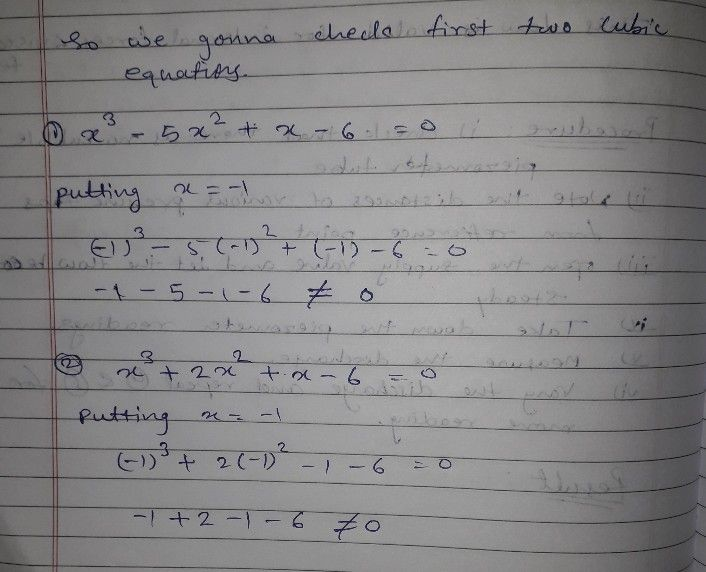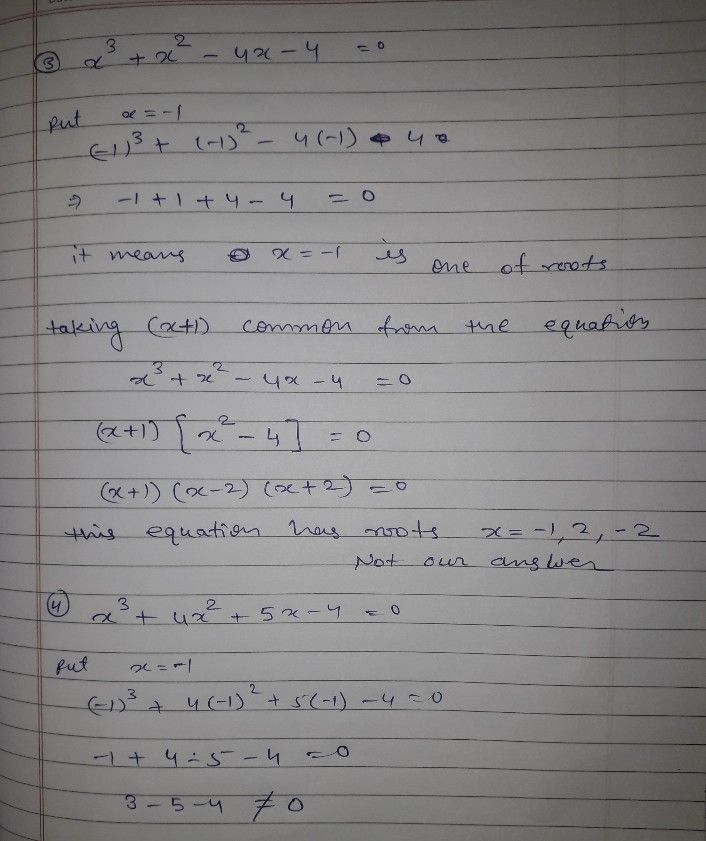Symbol
Problem$13$ Which polynomial equation has $a5$ $-1,2$ and $=3$ as its roots? E $\left(1$ Point) $○x\times 3-5xn2+x-6$ $○x^{n}3+2xn2+x-6$ $○x\times 3+xn2.4x.4$ $Ox^{n}3+4x^{n2+5x.4}$
Algebra
SolutionQanda teacher - Ankushh87one more option should be there.. None of these.
not a single equation has -3 as a root.